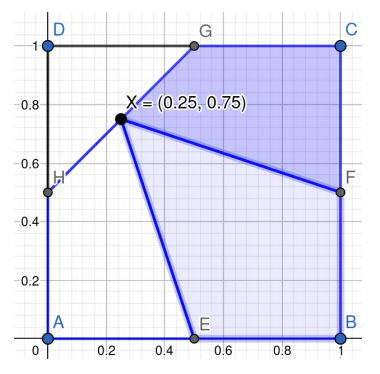시간 제한 메모리 제한 제출 정답 맞은 사람 정답 비율
1 초 1024 MB 0 0 0 0.000%

## 문제

David is a farmer and has a large farm. The shape of the farm is a square. A sqaure is a quadrilaterial that has four equal sides and four equal angles. The length of any side of David’s farm is one kilometer, so the area of his farm is slightly greater than the total area of 140 standard football fields.

David is old and very ill. He feels that his days are numbered. Worrying that his spouse Dora and their three children, Alice, Bob, and Cliff, will have a dispute over the ownership of the farm after he passes away, he plans to divide the farm into four parts, and then to allocate each part to one of his family members. He decides to write his last will as follows.

1. Assume that the shape of the farm is a sqaure ABCD where A = (0, 0), B = (1, 0), C = (1, 1), D = (0, 1).

2. Let E = (0.5, 0), F = (1, 0.5), G = (0.5, 1), H = (0, 0.5) be the midpoints of AB, BC, CD, DA, respectively.

3. Let area(PRQS) to denote the area of the quadrilaterial PQRS.

4. Please find a point X strictly inside the square ABCD such that

area(AEXH) : area(BF XE) : area(CGXF) = p : q : r

Note that X cannot be on the boundary of the square ABCD.

5. Allocate the land in AEXH, BF XE, CGXF, DHXG to Alice, Bob, Cliff and Dora, respectively.

David is still adjusting the numbers p, q, r, and his lawyer, Reed, has to read David’s last will carefully. Reed finds that it is impossible to find such point X if David gives an improper set of the numbers such as p = 1, q = 2, r = 1. However, there are proper sets of the numbers p, q, r that allow us to find the point X satisfying David’s last will. For instance, let p : q : r = 2 : 3 : 2, the following figure shows a possible position of X.Figure 2: area(AEXH) : area(BF XE) : area(CGXF) = 2 : 3 : 2

Please write a program to help Reed to determine whether it is possible to find a point X satisfying David’s last will for a given set of numbers p, q, r. If possible, please output one possible position of X to Reed.

## 입력

The input contains one line only. The line contains three space-separated positive integers p, q, r.

## 출력

If there does not exist a point X satisfying David’s last will, then output -1. Otherwise, output two irreducible fractions x and y such that (x, y) can be the point X satisfying David’s last will. You must output an irreducible fraction t = n d as n/d with a positive denominator and use exactly one space to separate x and y.

Note: the numerator and the denominator of any irreducible fraction are integers and do not have common divisors other than 1 and −1.

## 제한

• p, q, r ∈ {1, 2, . . . , 106}

## 예제 입력 1

1 1 1


## 예제 출력 1

1/2 1/2


## 예제 입력 2

1 2 1


## 예제 출력 2

-1


## 예제 입력 3

2 3 2


## 예제 출력 3

1/4 3/4